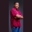Related Tags

sphere
volume
communitycreator
c#

# How to calculate the volume of a sphere in C#Theodore Kelechukwu Onyejiaku

### Overview

The capacity of a sphere is known as the volume of that sphere. In other words, it is the space occupied by the sphere. In C#, we can easily find the volume of a sphere.

### Syntax

4.0 / 3 * Math.PI * r * r * r
Syntax for calculating the volume of a sphere in C#

### Parameter values

Math.PI: This is an inbuilt value for the mathematic PI in C#.

r: This is the radius of the given sphere.

### Example

using System;
class HelloWorld
{
// create function to get the volume
static void GetVolume(double r){
double volume = 4/3 * Math.PI * r * r * r;
Console.WriteLine("The volume of the sphere with radius "+r+" is "+volume);
}

// main method
static void Main()
{
// create radius of some spheres
double r1 = 10;
double r2 = 1.5;
double r3 = 4.5;
double r4 = 8;

// get the volume of the spheres
GetVolume(r1);
GetVolume(r2);
GetVolume(r3);
GetVolume(r4);
}
}
Calculate the volume of a sphere in C#

### Explanation

• Line 5: We create a method called the GetVolume() method. This method takes the radius of the given sphere and calculates its volume. Then, it prints the result to the console screen.
• Lines 14–17: In the main method, we create the radius of some of the spheres whose volumes we want to find.
• Lines 20–23: We invoke the GetVolume() method and display the results on the console screen.

RELATED TAGS

sphere
volume
communitycreator
c#

CONTRIBUTORTheodore Kelechukwu Onyejiaku
RELATED COURSES

View all Courses

Keep Exploring

Learn in-demand tech skills in half the time